Concepts

Class 9
Chapter 4 Class 9 - Structure of Atom

## What are Valence Electrons?

• The electrons present in the outermost shell of an atom are called valence electrons.
• And the shell that contains the valence electrons is called the valence shell .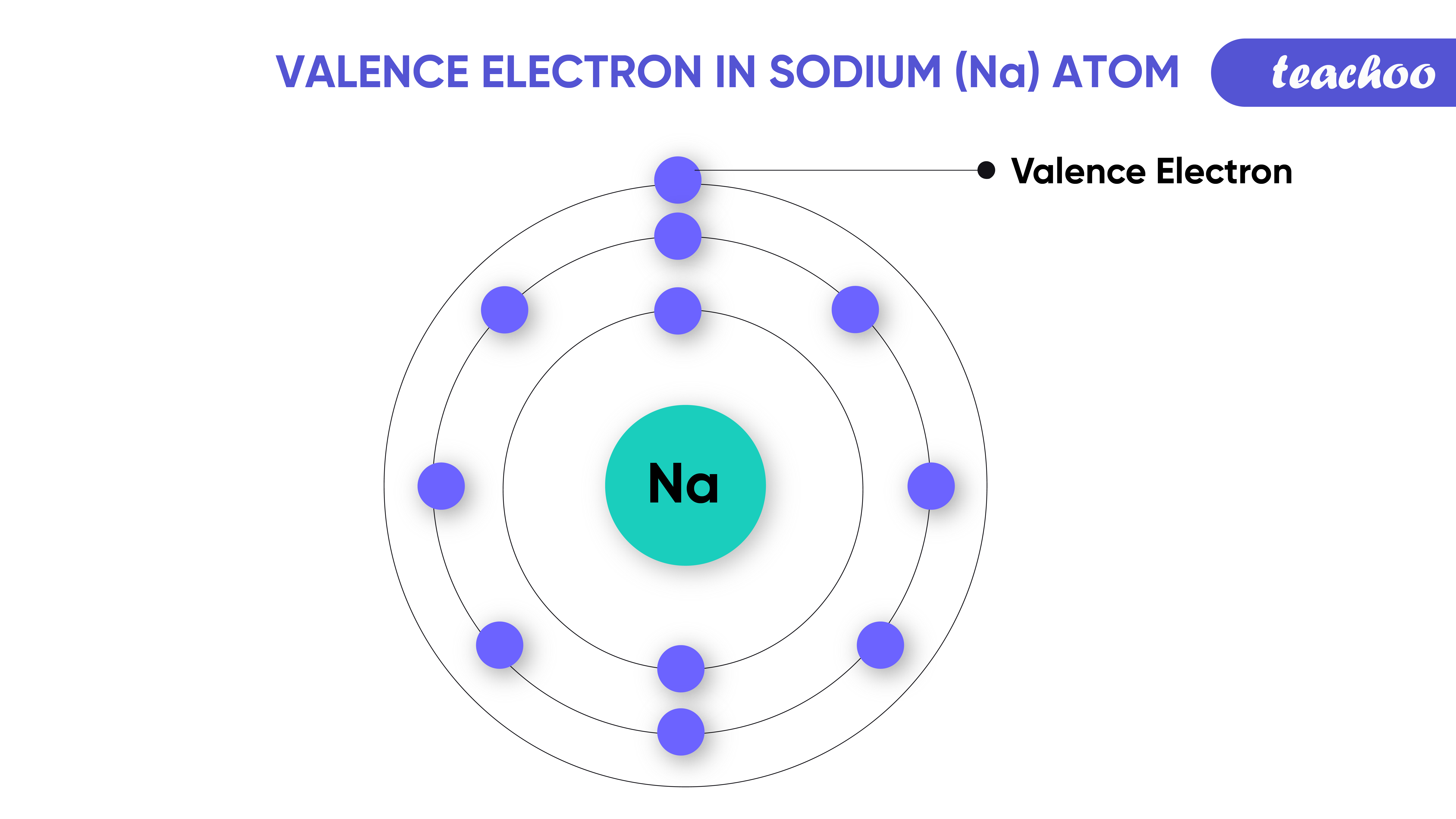## Example - 1

• Helium has 2 Electrons.
• All these are in K Shell (as K Shell can hold max 2 electrons).
• Hence Valence Electrons in Helium are 2 (as K shell is the outermost shell).
• Therefore, K Shell is the valence shell.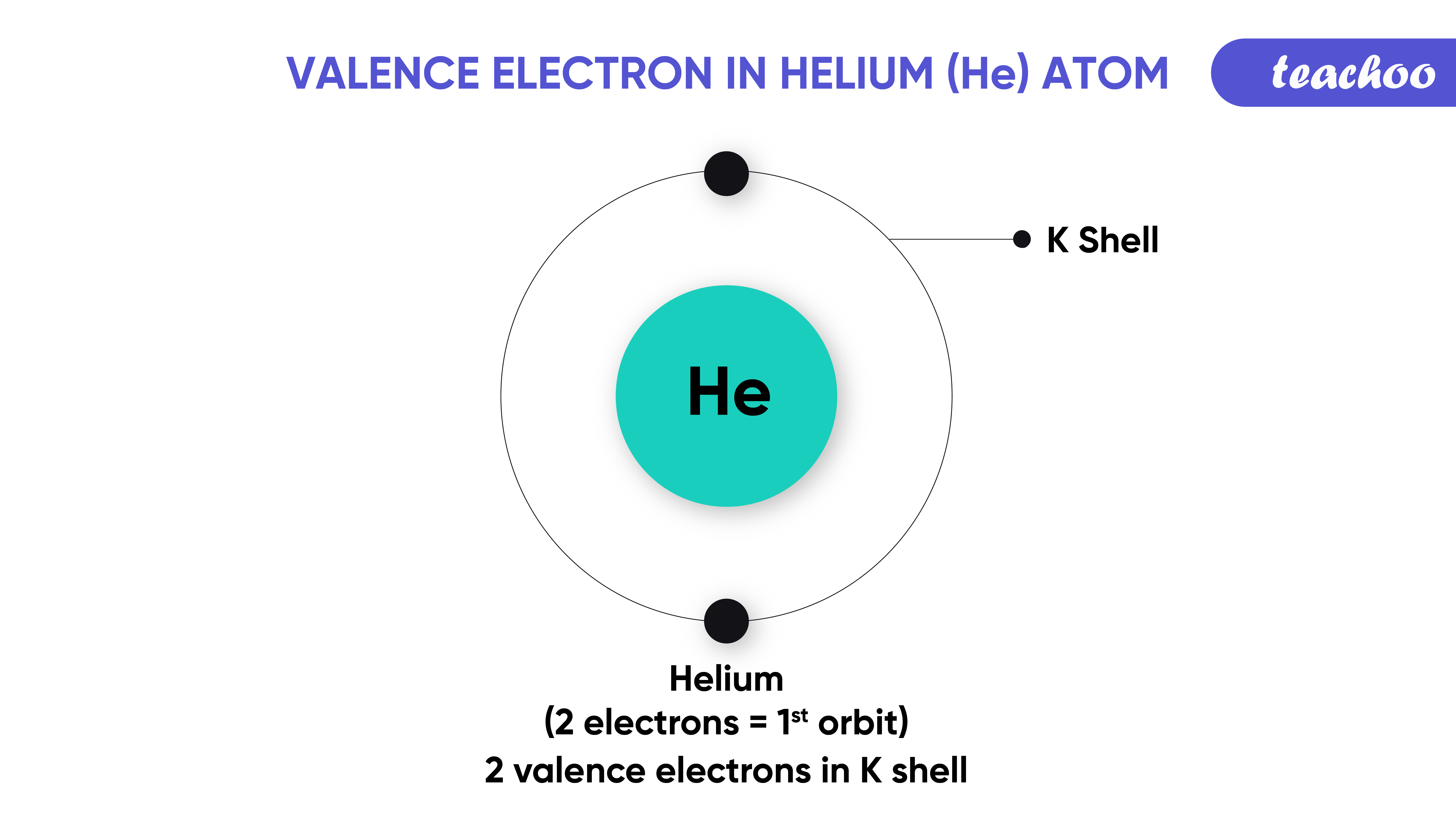## Example - 2

• Lithium has 3 Electrons.
• 2 of them are held in K Shell (as K Shell can hold max 2 electrons) .
• Remaining 1 is held in L Shell .
• Hence Valence Electrons in Helium is 1 (as L shell is outermost shell).
• Therefore, L Shell is the valence shell.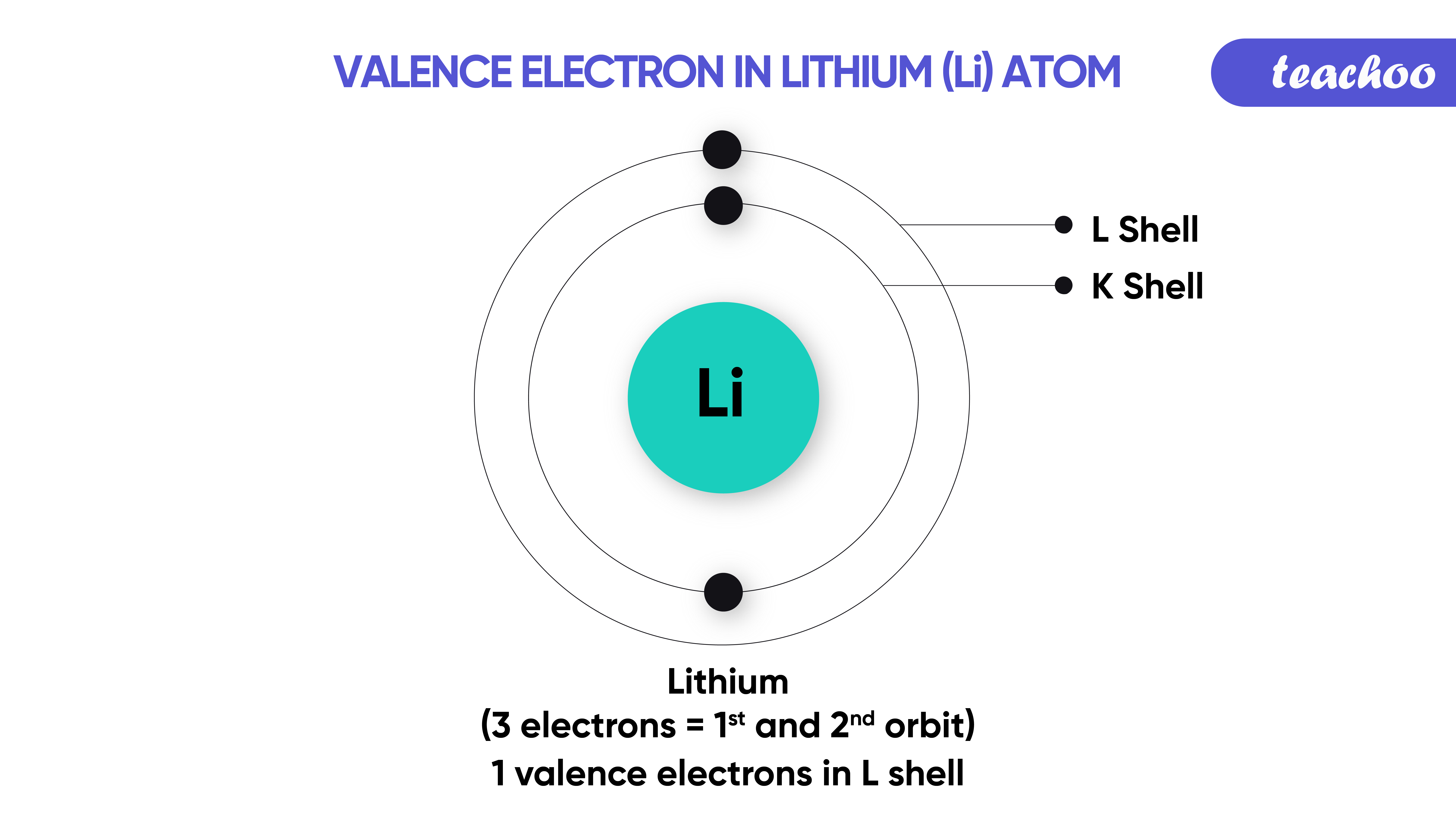## Example - 3

• Sodium has 11 Electrons.
• 2 of them are held in K Shell (as K Shell can hold max 2 electrons).
• 8 of them are held in L Shell (as L Shell can hold max 8 electrons).
• Remaining 1 is held in M Shell .
• Hence Valence Electrons in Sodium are 1 (as M shell is Outermost shell).
• And M Shell is the valence shell .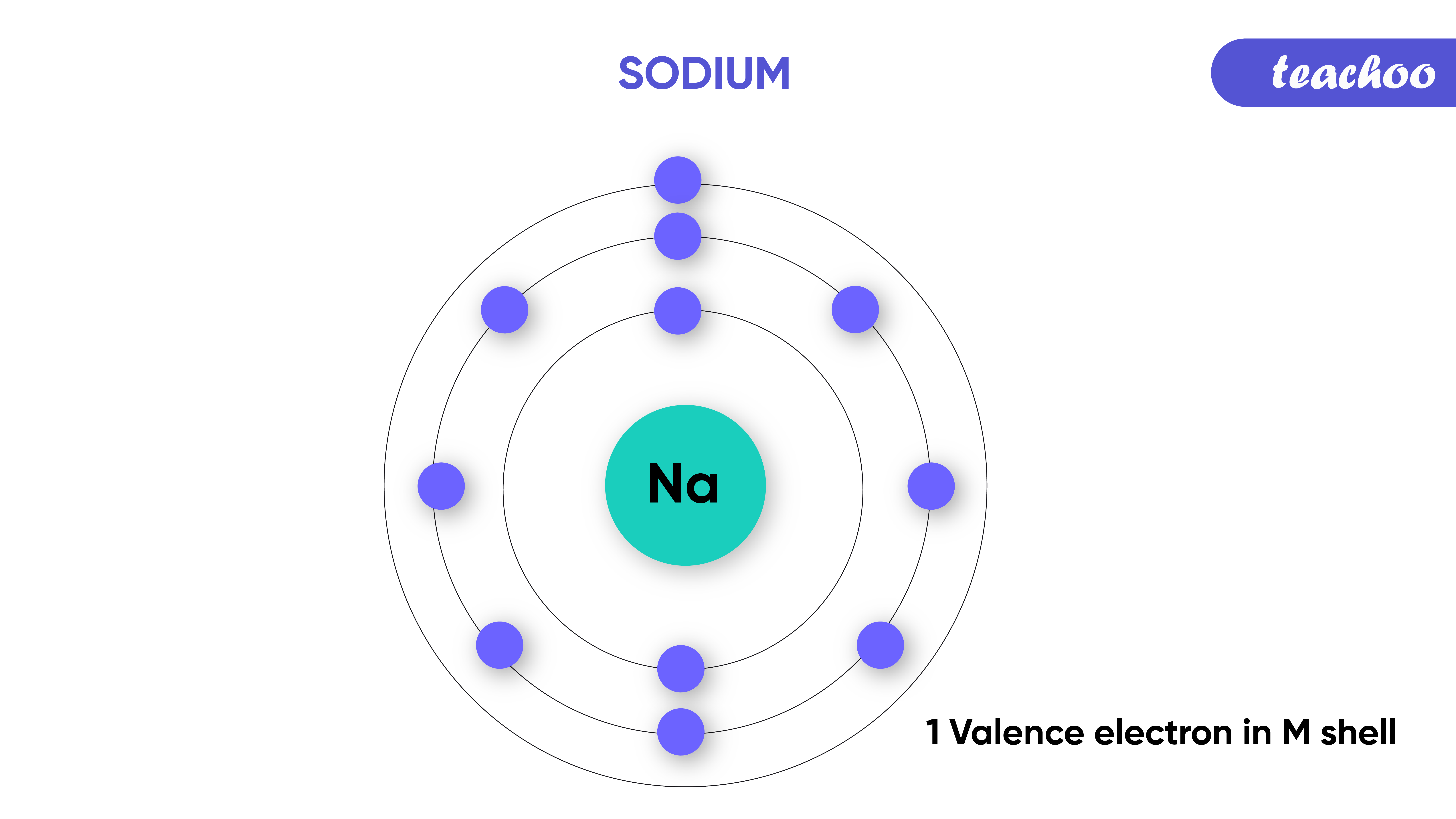## Why are Valence Electrons important?

• We know that valence electrons are the electrons present in the outermost shell of an atom.
• Only these electrons take part in chemical reactions .
• Those having their outermost shell full do not react with other elements

Example : -

• Helium has 2 Elements in Outer shell .

• All these are in K Shell (as K Shell can hold max 2 electrons).

• Hence, there are no free electrons which take part in chemical reactions .

• The outermost/valence shell of an atom can contain at-most 8 electrons only . If there are more than 8 electrons, then the remaining electrons will go to the next shell.
• This is known as the octet rule .

For example - Calcium has 20 electrons

Note that only the K shell has a maximum of 2 electrons , the remaining shells can accomodate 8 electrons each . After accommodating 8 electrons, the remaining electrons move to the next shell and so on.

Its electrons are divided in shells as -

K shell   2 electrons

L shell   8 electrons

M shell 8 electrons

N shell 2 electrons (the valence electrons)

Even though the M shell (n = 3) can contain 2n 2 = 2 x 3 2 = 2 x 9 = 18 electrons

• But since it will become the valence shell, it cannot accommodate more than 8 electrons.
• The remaining 2 e- will go to the N shell, making the N - shell to be the valence shell.

Note:- The atoms are most stable when they've 8 electrons in their valence shell.

Hence, elements generally combine in order to exchange electrons and achieve octet.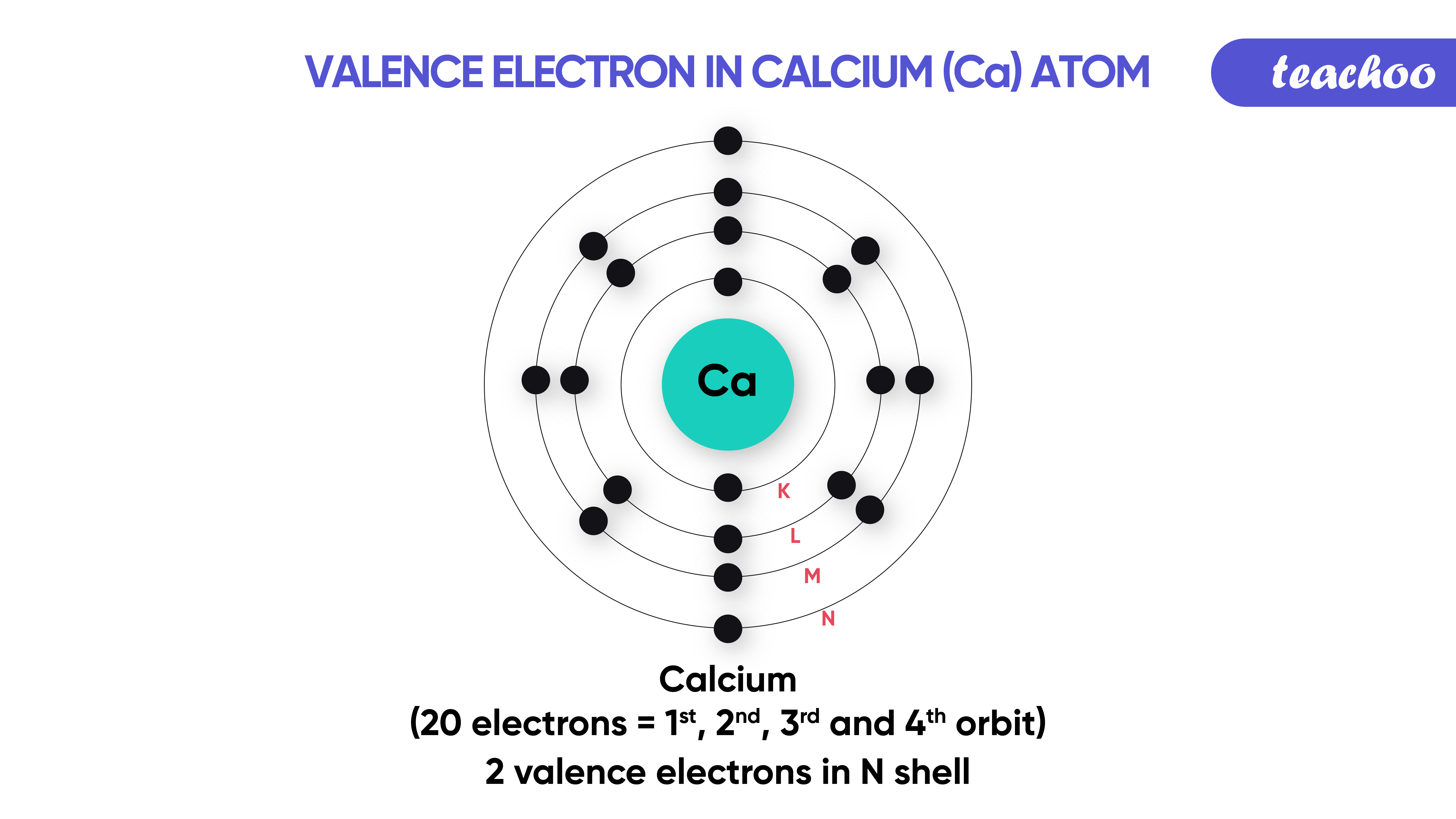Examples -

In text Question -

NCERT Back Exercise - Q17, Q18

## What is Valency ?

• It is the combining capacity of an element.

## Example:

• Valency of Hydrogen is 1.
• Valency of Oxygen is 2.
• Hence 2 atoms of Hydrogen are needed to combine with 1 atom of Oxygen to form 1 Atom of Water (H 2 O).

## How is Valency of An Element Determined?

• Valency depends upon Valence Electrons (Number of Elements in Outermost shells).
• We know that electrons are negatively charged particles.
• They revolve around the nucleus, which is a very small positive body.
• An atom is neutral because the positive charge of the nucleus is equal to the negative charge of electrons.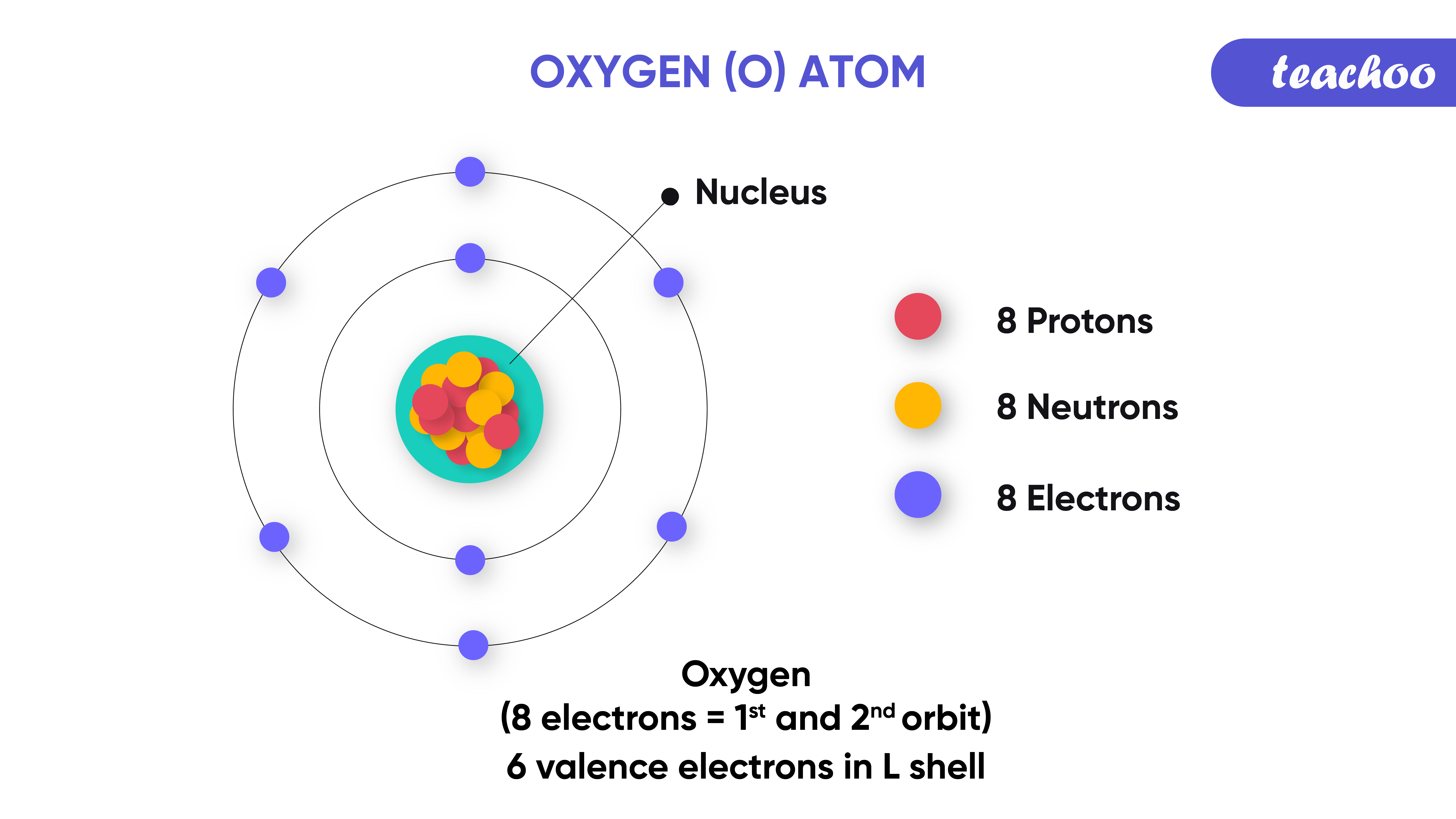## Now, how do we determine the valency of an atom?

• The maximum number of electrons in a valence shell can be 8.
• Hence, the number of electrons in a valence shell can vary from 1 to 8 .

1. Now, if an atom has 1, 2 or 3 number of valence electrons

It is easier for them to lose 1, 2 or 3 e- respectively from their valence to achieve an octet.

(Since now the previous shell would be its valence shell, containing 8 electrons)

Valency of atoms losing 1 electron = 1

Valency of atoms losing 2 electrons = 2

Valency of atoms losing 3 electrons = 3

## Example - 1

• Sodium has a total 11 Electrons.
• 2 of these are held in K Shell (as K Shell can hold max 2 electrons).
• 8 of these are held in L Shell (as L Shell can hold max 8 electrons).
• Remaining 1 is held in M Shell .
• Thus Sodium needs to lose 1 electron to achieve octet (8 Electrons in the outermost shell).
• Hence, Valency of Sodium is 1.## Example - 2

• Magnesium has a total 12 Electrons.
• 2 of these are held in K Shell (as K Shell can hold max 2 electrons).
• 8 of these are held in L Shell (as L Shell can hold max 8 electrons).
• Remaining 2 are held in M Shell .
• Thus Magnesium needs to lose 2 electrons to achieve an octet (8 Electrons in the outermost shell).
• Hence Valency of Magnesium is 2.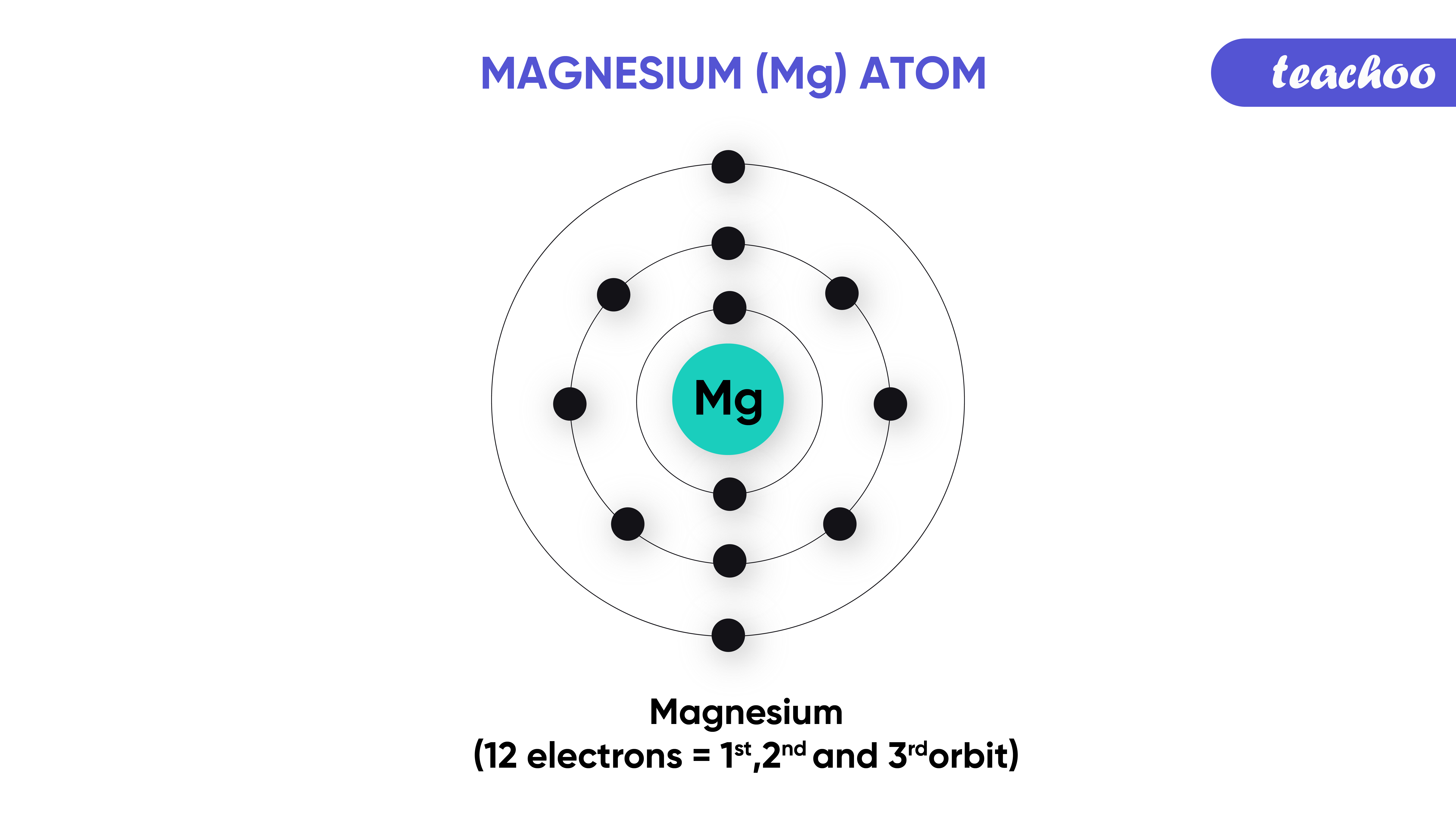2.    If an atom has 5, 6 or 7 number of valence electrons.

It is easier for them to gain 3, 2 or 1 electron respectively in their valence to achieve an octet.

(Since after gaining 3, 2 and 1 electron respectively, their valence shell will have 8 electrons).

Valency of atoms gaining 1 electron = 1

Valency of atoms gaining 2 electron = 2

Valency of atoms gaining 3 electron = 3

## Example - 1

• Oxygen has a total 8 Electrons.
• 2 of these are held in K Shell (as K Shell can hold max 2 electrons).
• Remaining 6 are held in L Shell.
• Hence Valence Electrons in Oxygen are 6 (as L shell is outermost shell).
• Thus Oxygen needs to gain 2 electrons to achieve an octet (8 Electrons in the outermost shell).

Hence Valency of Oxygen is 2.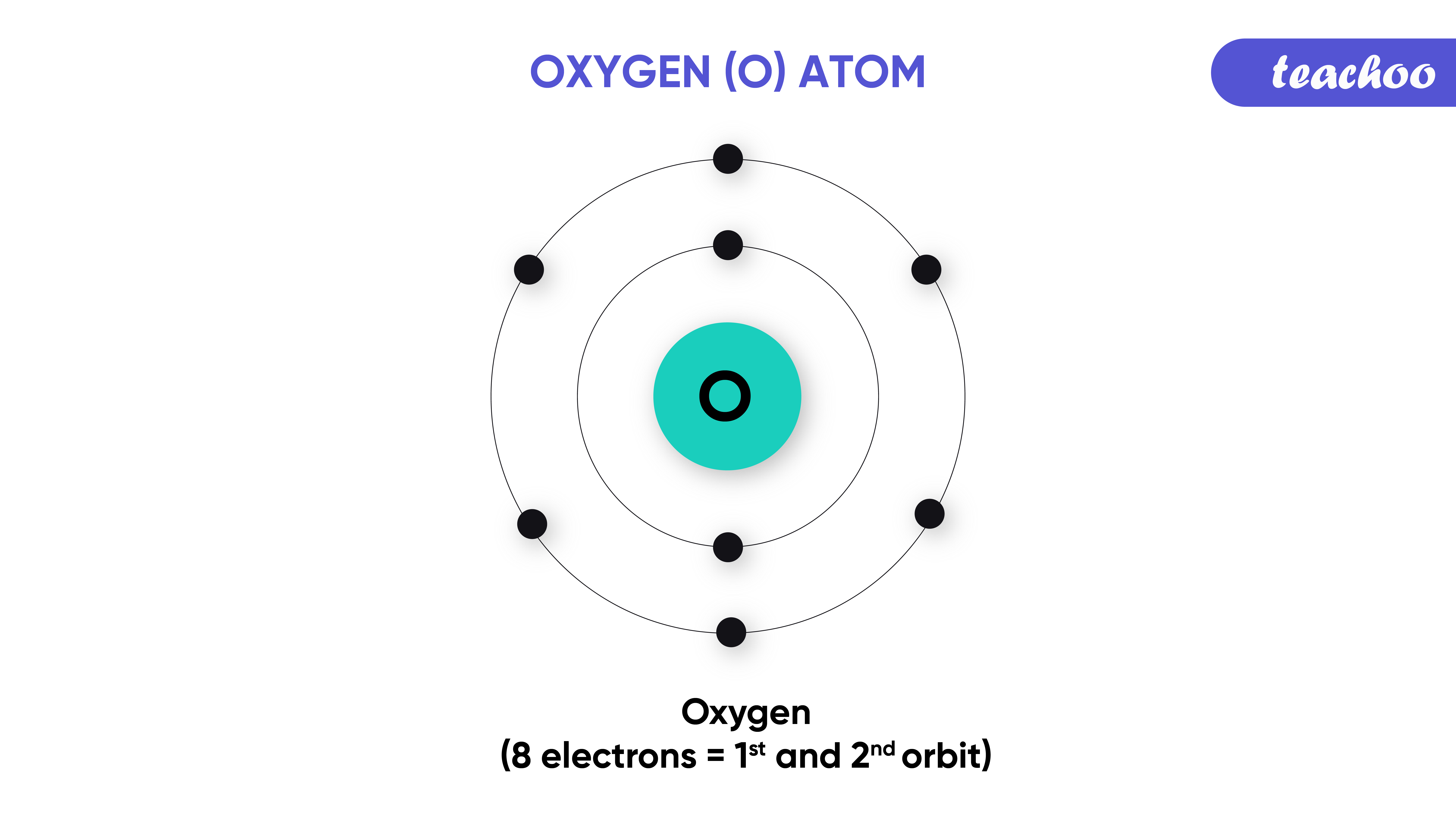## Example - 2

• Nitrogen has a total 7 Electrons.
• 2 of these are held in K Shell (as K Shell can hold max 2 electrons).
• Remaining 5 are held in L Shell.
• Hence Valence Electrons in Nitrogen are 5 (as L shell is outermost shell).
• Thus Nitrogen needs to gain 3 electrons to achieve octet (8 Electrons in outermost shell).
• Hence Valency of Nitrogen is 3.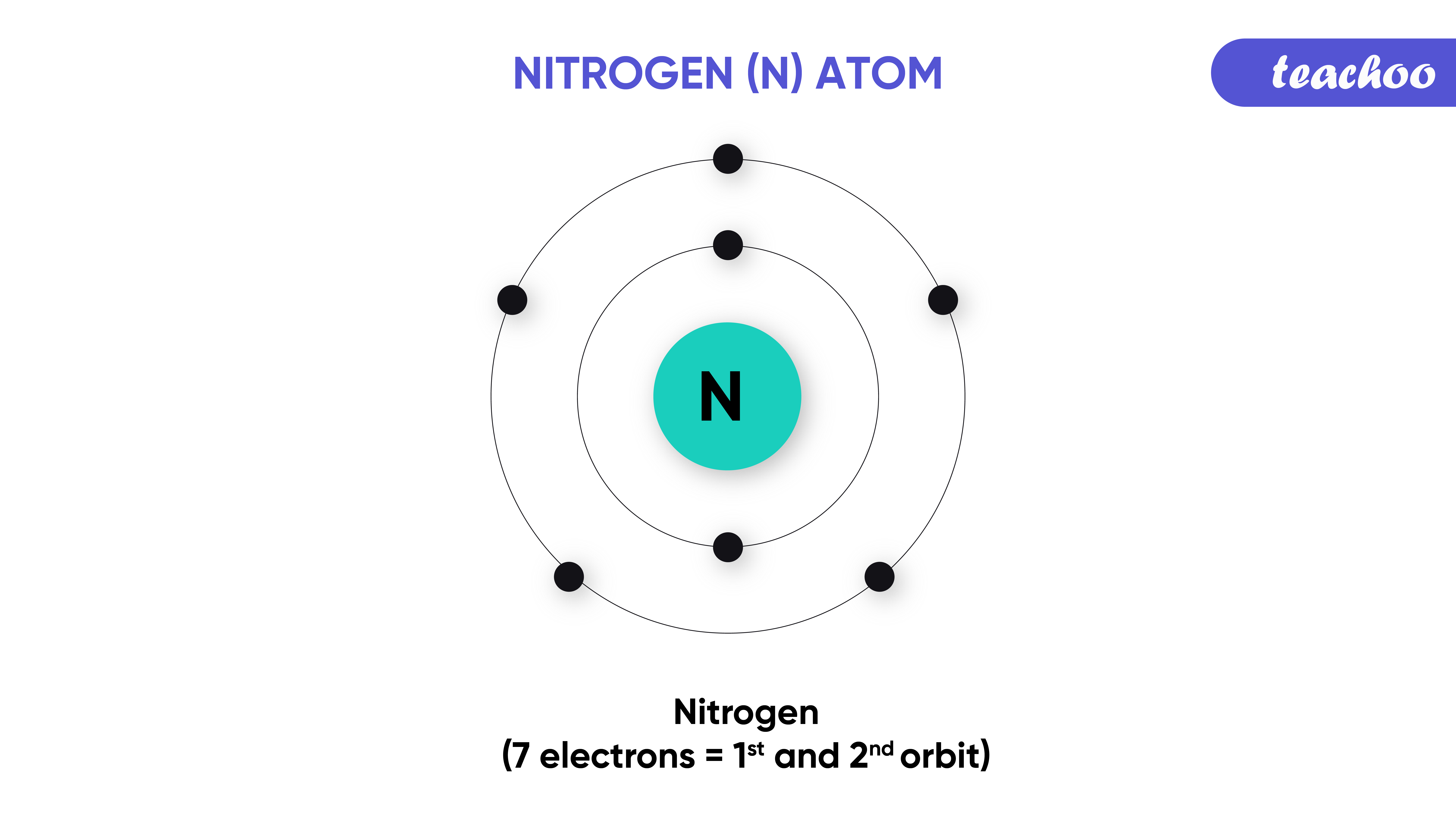3.  If an atom has 4 electrons in its valence shell , then it has an equal chance of gaining, losing or sharing it's valence electrons in order to  achieve octet.

In any case, the valency of such atoms is 4

## Example - 3

• Silicon has a total 14 Electrons.
• 2 of these are held in K Shell (as K Shell can hold max 2 electrons)
• 8 are held in L Shell .
• The remaining 4 are held in the M shell.
• Hence Valence Electrons in silicon are 4 (as L shell is outermost shell).
• Thus silicon needs to gain 4 electrons or lose 4 electrons to achieve octet (8 Electrons in the outermost shell).
• Hence, the Valency of silicon is 4.

Note: - If an atom has 8 electrons in its valence shell

Then it has already achieved octet and is stable

Hence, it won't lose, gain or share electrons.

Their valency will be 0.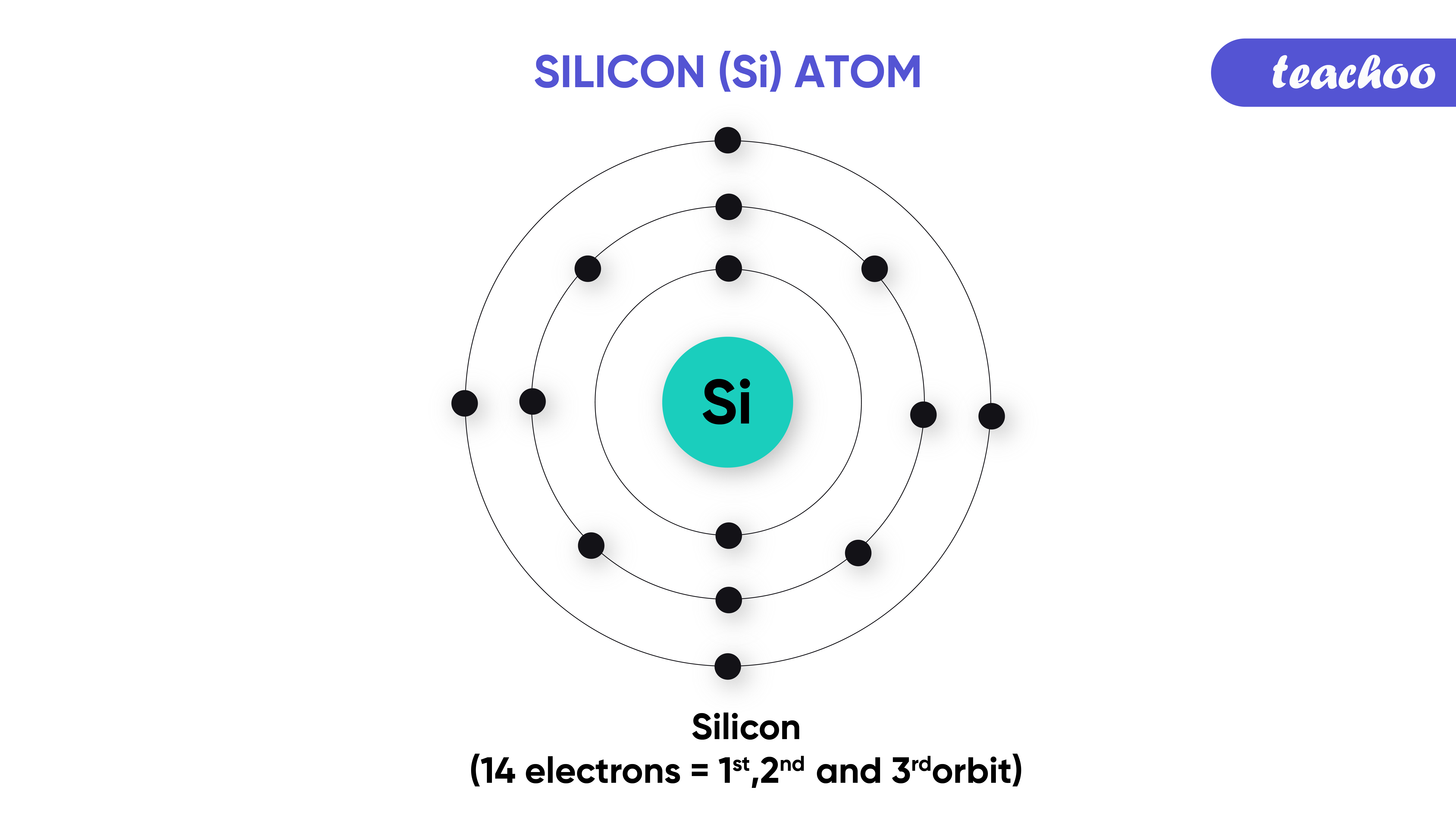Examples -

In text Question - Page 52 Q1 Left

NCERT Back Exercise - Q7

## What are Inert Gases or Noble Gases?

• They are those gases which do not combine with other elements to form compounds.
• Valency of these elements is 0.

Different Noble Gases are: -

 Element Atomic Number Valency Helium 2 0 Neon 10 0 Argon 18 0 Krypton 36 0 Xenon 54 0 Radon 86 0

## Why do the Noble Gases not react?

• It is because the electron arrangement in these gases is very stable.
• These normally have 8 electrons in their outermost shells (except helium).
• Hence, they do not need to gain or lose electrons to achieve an octet.

## Example - 1

• Neon has total 10 Electrons
• 2 of these are held in K Shell (as K Shell can hold max 2 electrons).
• 8 of these are held in L Shell (as L Shell can hold max 8 electrons).
• Hence in the outermost shell, already octet (8) achieved.
• It also means they do not need to gain or lose electrons to achieve an octet.
• Hence, Valency of Neon is 0 and it is called inert gas or noble gas .## Example - 2

• Argon has 18 electrons
• 2 of these electrons are held in K Shell (as K Shell can hold max 2 electrons).
• 8 of these electrons are held in L Shell (as L Shell can hold max 8 electrons).
• Remaining 8 electrons are held in M Shell .
• Hence in the outermost shell, already octet (8) achieved.
• It means they do not need to gain or lose electrons to achieve an octet.
• Hence, Valency of Argon is 0 and it is called inert gas or noble gas.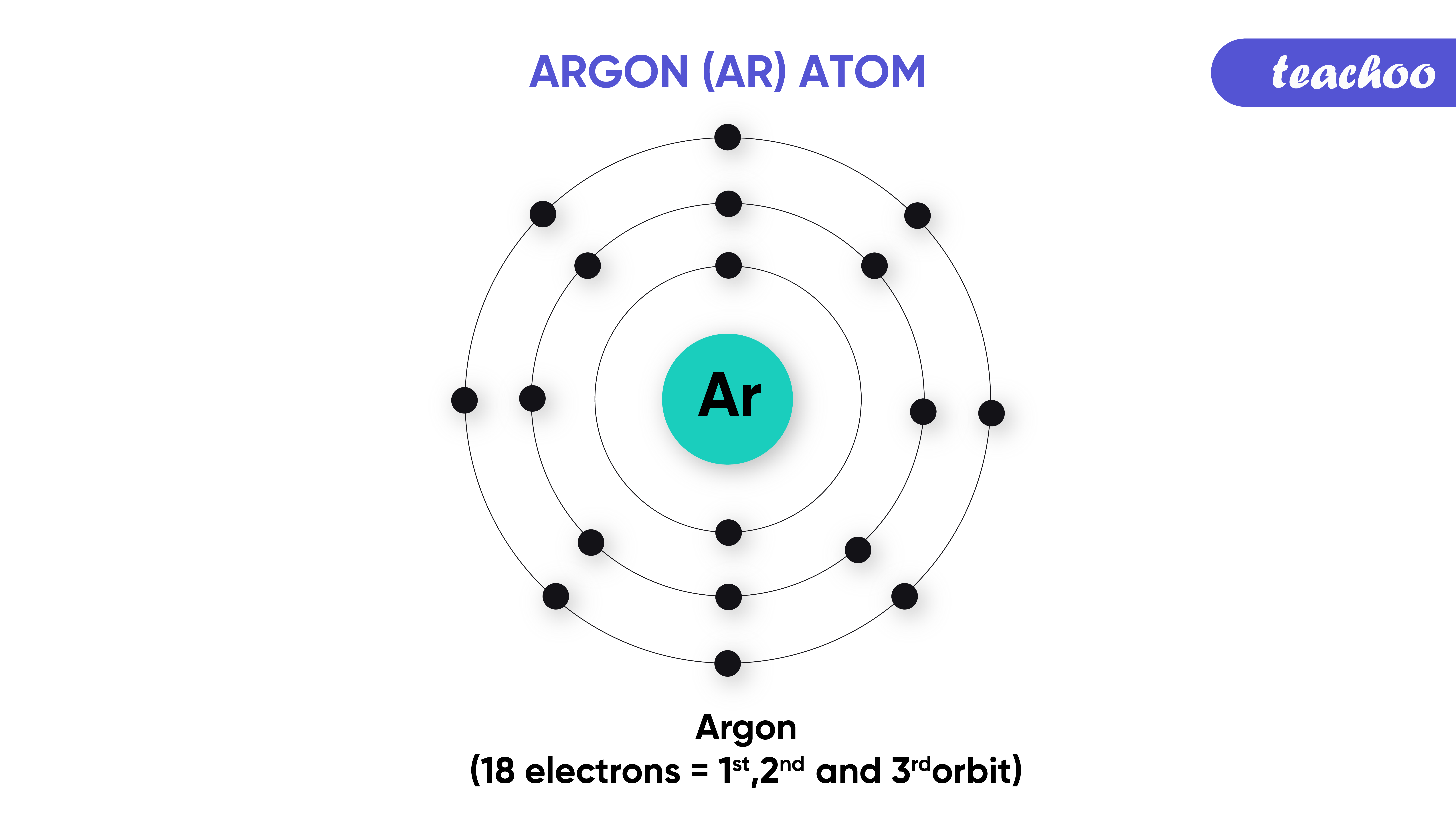Note:-

All Noble Gases Achieve Octet except Helium

Examples -

In text Question -

NCERT Back Exercise -

Example 3

• Helium has 2 Electrons.
• All these are in K Shell (as K Shell can hold max 2 electrons).
• Hence the outermost shell is the K shell which is already full.
• Now, they do not need to gain or lose electrons.
• Hence, Valency of Helium is 0 and it is called inert gas or noble gas .

Introducing your new favourite teacher - Teachoo Black, at only ₹83 per month